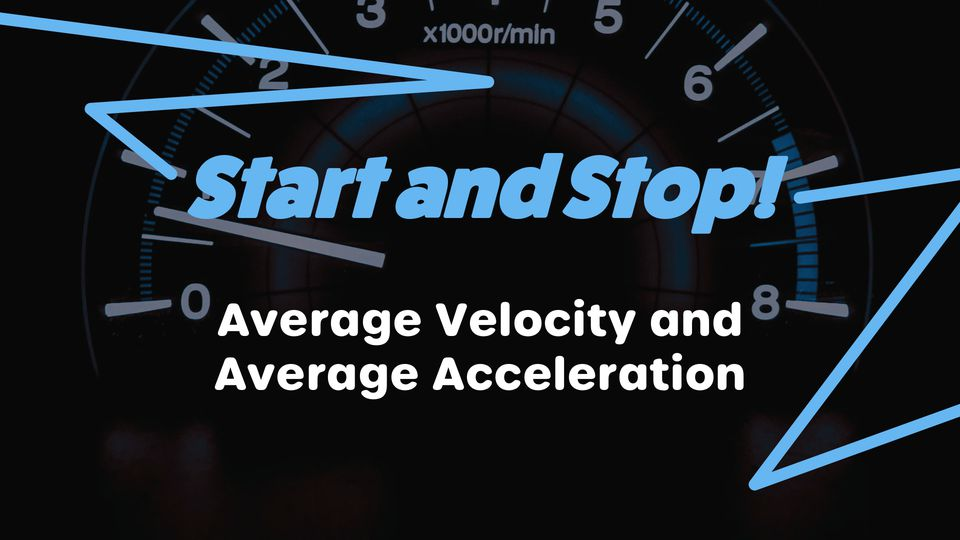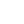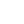Preview the Course
1h 8m

# Average Acceleration and Velocity Formula

by Robert Abramovic | Science FREE6 Lessons 1:08:28
1.1. Introduction 6:29
2.2. Instantaneous Rate of Change 9:26
3.3. Average Rate of Change 13:02
4.4. Average Rate of Change vs. Instantaneous Rate of Change 17:19
5.5. Average Velocity 11:43
6.6. Average Acceleration 10:29

1h 8m

NGSS Standards

FREE

Course

Computing the average acceleration and velocity formula is a simple physics experiment anyone can do. All one needs to do is take measurements of the same quantity at two recorded times, take the difference between those two measurements, and divide by the difference between the two times. In this course, you will learn the difference between the instantaneous rate of change and the average rate of change. You will learn how the definition of average velocity and average acceleration as well as how to compute each. Examples of a car driving on a freeway and taking a look at the speedometer or odometer reading two separate times are presented to make the concepts relatable.

Tags:

• Acceleration
• Kinematics
• Math
• Physics
• Science
• STEM
• Velocity

## Instructor

Hi, I'm Robert. I hold a Ph.D. in Mathematics as well as a BA in Physics. I have been a private tutor for over 13 years. I enjoying sharing my knowledge of mathematics and physics with students, creating relatable examples, and making learning fun. My philosophy is not only teaching students but inspiring them to learn more about the material on their own.

See full profile